# How to convert a string to float in JavaScript – The Ultimate Tutorial

In this tutorial, let’s look at the different ways to convert a string to a floating-point number. We’re not just going to stop after looking at the obvious parseFloat method.

We’re going to delve further into the different ways to use the common JavaScript operations and methods to achieve the same parsed result. Let’s get into it then!

## Using the parseFloat() method

The most straightforward way to convert a string to float in JavaScript is by using the parseFloat() method. As the name of the function implies, we can use it to parse a string and convert it into a floating-point number; that is, a number with decimal points.

You just need to call the method by sending the number as the first, and most times, the only argument.

Let’s start our example by creating a variable ‘num’ and assigning it a string value ’12.55’. Then, let’s parse the string through parseFloat.

```let num = '12.55';
console.log(typeof num);
num = parseFloat(num);
console.log(num);
console.log(typeof num);```

Let’s check the result and the type of the result, and we’ll get this: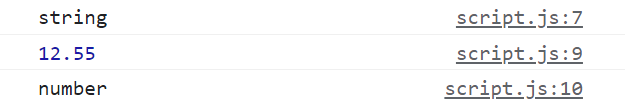## JavaScript string to float 2 decimal places

You can go a bit further and specify the number of decimal places you want in your floating-point number as well.

To do that, use the toFixed method on the parsed number, and give the number of decimal points you need as the argument within the brackets, like shown below.

```let num = '12.5479257';
num = parseFloat(num).toFixed(2);
console.log(num);```

Run the above snippet, and you’ll get the following output: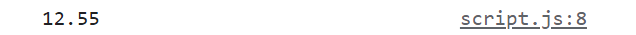As you can see, our number has been truncated to just 2 decimal points, and the function went ahead and rounded up our last decimal place for us as well.

That’s great indeed, but if you check the type of this number now, you won’t get ‘number’ as the result. You’ll get ‘string’. Why is that?

Well, the toFixed() method does fix the decimal places, but it also converts the number back to a string while doing that. So unfortunately, if you’d like to use the number in any operation, you must convert it back to a floating-point number by using the parseFloat method, as shown below:

```let num = '12.5479257';
num = parseFloat(num).toFixed(2);
console.log(`Number is : \${num}`);
console.log(`Type of num after toFixed: \${typeof num}`);
num = parseFloat(num);
console.log(`Type of num after parseFloat: \${typeof num}`);```

Now, run the entire snippet to get the following output: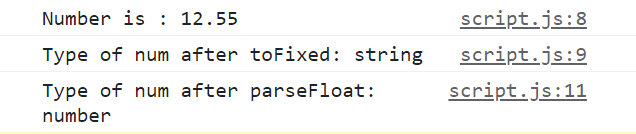## Multiply with a number

ParseFloat is great, as well as straightforward. But, if you’d like to avoid pre-defined JavaScript methods, you can just use one of the mathematical operations in JavaScript, especially the multiplication operation, to convert your string to a number, be it integer or floating point, as shown below:

```let num = '12.54';
num = num * 1;
console.log(typeof num);```

When you run the above program, you’ll get: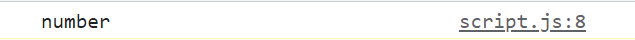As you can see, the string has been successfully converted to a number, from a simple multiplication operation.

Why does this happen? Well, since multiplication is not string concatenation (string concatenation can be done with only the ‘+’ and ‘+=’ operators), multiplication does a simple type casting, and converts your string to a number, especially if your browser sees that the string is just numbers within quotes.

Note that this method does not work if you have just a bunch of letters (characters) inside of your string. If that’s the case, you’ll end up with NaN (Not a Number) as the result.

If you multiply the string by 1, you’ll convert the string to a number, while still retaining the value.

You can achieve the same with an integer number as well, by just placing an integer value inside a string, as shown below:

```let num = '12';
num = num * 1;
console.log(typeof num);
console.log(num);```

The type of the resultant value would be a ‘number’ and the number in itself would be the integer 12, as seen in the output shown below: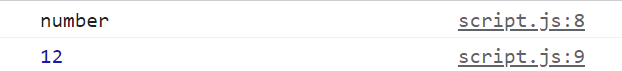If it’s a whole number, it won’t add trailing zeros though, as you just saw. This is true for parseFloat as well, since in JavaScript, for numbers, there can’t be trailing zeros.

```let num = '12.00';
num = parseFloat(num);
console.log(`Result after parseFloat on 12.00 is : \${num}`);```

We still get only 12, and not 12.00, as shown below: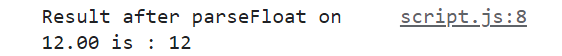## Implicit type casting with a unary plus

If you’d like to keep things simple, go for a simple type casting where the string is automatically converted to a number, and in our case, a decimal number, because the unary plus in front of it forced our browser to do so.

Look at the example below:

```let num = '12.567';
num = +num;
console.log(`Type of value after unary + on 12.567 is : \${typeof num}`);```

As you can see, we’ve just placed a unary plus before ‘num’ and reassigned it back to ‘num’, so we can get the number form of the string. Now, if we check the type of the newly assigned value, we’ll get: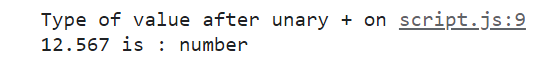## The Number() method to convert a string to float

Using the unary plus is great because you get a type conversion while the value does not change at all. But why don’t we just use the straightforward Number() method that converts a string to a number, no matter what the value is.

If your string starts with a bunch of characters (or only has characters), then it’ll just return a NaN value, otherwise, you’ll get back a number. If your string has decimal numbers in it, you’ll get a floating value; otherwise, you’ll end up with an integer value. It’s as simple as that.

Use the number method, and send over the string you want converted as the argument, and you’re done, as shown below:

```let num = '12.567';
num = Number(num);
console.log(typeof num);```

If you run the above lines of code, you’ll get back a ‘number’ result for the variable ‘num’ now.

## Handle numbers with commas

So far, we’ve looked at different ways to handle type conversions between strings and floating-point numbers, and they were very straightforward methods. We had a number, and we converted, it. That’s it.

But, in the real-world, people tend to add characters to numbers, especially commas. What if your number had commas? If you try to use a parseFloat on the exact number (with all its commas), a good portion of your number would be left out of the conversion. We’ll talk about that a little further in the article.

For now, just know that you should handle the commas before you let them pass through the parseFloat method. The best way to remove your commas is by using the string replace method and the power of regular expressions.

Look at the example below:

```let num = '12,543.456';
num = parseFloat(num.replace(/,/g,''));
console.log(num);```

In the above example, we’re using the string replace method, and we’re sending ‘,’ as the regular expression. We’re also using the global flag (g) to make sure that the program catches all the instances of commas in the string.

We’re replacing all the commas with an empty string, and then we’re pushing the new string (number without commas) into the parseFloat method.

The result would be 12543.456, a floating-point number with 3 decimal places.

## More examples with ParseFloat()

As I told you before, we have the means to handle strings within numbers, if that’s all they have. What if they have characters in between, or if the string is made up of only characters (and has no numbers in it)?

We’ve already looked at how to handle commas in-between our numbers, mostly because we’ll still end up with a legitimate number once we remove them. But what if the characters are random? What does the parseFloat method do then? Let’s look at a bunch of examples.

If your string has numbers, followed by characters, be it letters, spaces or special characters, parseFloat will just ignore the leading characters and just convert the number.

```let num = '12xy ()';
num = parseFloat(num);
console.log(`PaseFloat on '12xy ()' is : \${num}`);```

When you run the above line of code, you’ll just get the number 12 as the result, as shown below: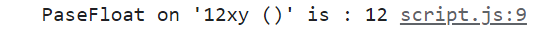### Strings with leading or trailing spaces

If the number has leading or trailing spaces, or both, and no other special characters, you’re in luck. parseFloat() will just ignore the spaces, and return the number, and that’s it.

`let num = '   10   ';`

The above will just return:### Strings with characters followed by numbers:

If you have characters followed by numbers, and not the other way around though, you’re out of luck.

You’re going to get back a NaN, which means ‘Not a Number’, because your browser can’t look beyond those first characters in the string. The numbers don’t matter in this case.

`let num = 'ag10';`

So, the above string will send back: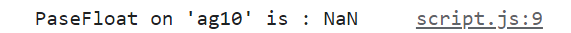### Strings with numbers followed by characters, and then again numbers:

In some instances, just like we saw with commas, you might have random characters between your numbers. If you can replace them beforehand like we did with commas, we can still extract the full number.

In some cases, you can’t replace the characters beforehand because they’re random. When that happens, parseFloat() will just extract the numbers at the start of the string, right before the characters start, and ignore the rest (numbers and characters alike).

`let num = '10ag10';`

When we run the above lines of code, we’ll get: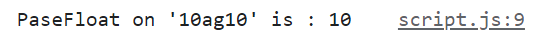### Strings with 2 decimal places:

If a string has numbers with 2 decimal points, the second decimal point, and the numbers after that will be ignored.

`let num = '10.45.65';`

Run the above lines of code, and you’ll get: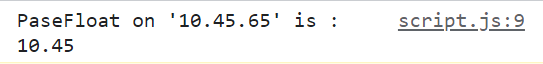## Check beforehand with isNaN()

Generally, in a real-world program, right after you convert a string to a floating-point number, you’ll have a calculation lined up for that number.

In that case, it’s better to check if the value you got back after the conversion is a number or a NaN value. This will create a checkpoint that’ll save you a lot of headaches down the line.

The best way to check if a value is NaN is by using the isNaN method. It’s a pre-defined JavaScript method and returns true if the value is NaN and false if the value is a number.

In the below example, we’re created a checkpoint that assigns 0 to num if the parseFloat of num returns NaN. If not, it assigns the type converted value to num. Finally, it prints the result.

```let num = 'xc3';
if(isNaN(parseFloat(num))) {
num = 0;
}

else {
num = parseFloat(num);
}

console.log(num);```

There you go! This was a jam-packed tutorial.

We looked at 4 different ways to convert a string to float in JavaScript,

1. By using the parseFloat method
2. By multiplying the string with 1
3. By using Unary plus
4. By using the Number() method

Apart from these 4 methods, we also looked at

• How the parseFloat method performs in different scenarios,
• How to use the toFixed() method to trim the decimal places, and
• How to place a checkpoint that checks if the result of the conversion is NaN before (to avoid errors or miscalculations down the line).